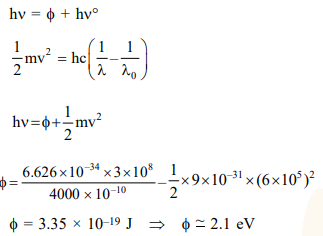Deepak Scored 45->99%ile with Bounce Back Crack Course. You can do it too!

# What is the work function of the metal if the light of wavelength

Question:

What is the work function of the metal if the light of wavelength $4000 \AA$ generates photoelectrons of velocity $6 \times 10^{5} \mathrm{~ms}^{-1}$ form it ?

(Mass of electron $=9 \times 10^{-31} \mathrm{~kg}$

Velocity of light $=3 \times 10^{8} \mathrm{~ms}^{-1}$

Planck's constant $=6.626 \times 10^{-34} \mathrm{Js}$

Charge of electron $=1.6 \times 10^{-19} \mathrm{JeV}^{-1}$ )

1. $0.9 \mathrm{eV}$

2. $4.0 \mathrm{eV}$

3. $2.1 \mathrm{eV}$

4. $3.1 \mathrm{eV}$

Correct Option: , 3

Solution: